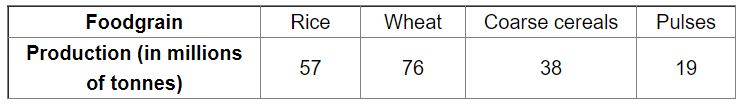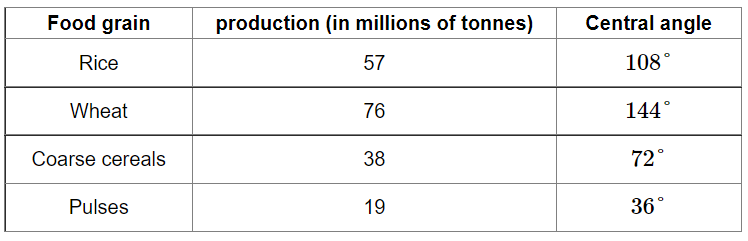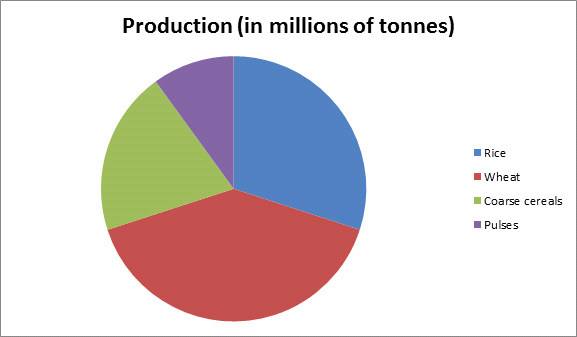# The following data shows the agricultural production in India during a certain year.

Question:

The following data shows the agricultural production in India during a certain year.Draw a pie chart to represent the above data.

Solution:

Total production $=(57+76+38+19)=190$

Central angle of each foodgrain $=\left(\frac{\text { production of each foodgrain }}{\text { total production }} \times 360\right)^{\circ}$

Calculation of central anglesConstruction of pie chart

Steps of construction:

1. Draw a circle of any convenient radius.

2. Draw a horizontal radius of the circle.

3. Draw sectors whose central angles are $108^{\circ}, 144^{\circ}, 72^{\circ}$ and $36^{\circ}$.

4. Shade the sectors so obtained differently and label each one of them.

Thus, we obtain the required pie chart as shown in the figure below.